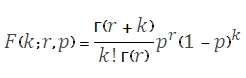# SQL Server NEGBINOM.DIST function

Updated: 9 August 2010

Use NEGBINOMDIST to calculate the negative binomial distribution. NEGBINOMDIST returns the probability that there will be k failures before the rth success, when the constant probability of a success is p. The formula for the negative binomial distribution is:Where
k              is the number of failures
r              is the number of successes
p             is the probability of a success

Syntax
SELECT [wctStatistics].[wct].[NEGBINOMDIST] (
<@Number_f, float,>
,<@Number_s, float,>
,<@Probability_s, float,>)
Arguments
@Number_f
is the number of failures. @Number_f is an expression of type float or of a type that can be implicitly converted to float
@Number_s
is the number of successes. @Number_s is an expression of type float or of a type that can be implicitly converted to float
@Probability_s
is the probability of a success. @Probability_s is an expression of type float or of a type that can be implicitly converted to float
Return Types
float
Remarks
·         If @Probability_s <0 or @Probability_s > 1, NEGBINOMDIST returns an error.
·         If @Number_f < 0 or @Number_s < 1, NEGBINOMDIST returns an error.
·         NEGBINOMDIST = BINOMDIST(@Number_s,@Number_f+@Number_s,@Probability, 'False')*@Number_s/(@Number_f+@Number_s)
Examples

SELECT
wct.NEGBINOMDIST(4,90,0.975)

This produces the following result
----------------------
0.116820452331376

(1 row(s) affected)

SELECT
wct.BINOMDIST(90,90+4,0.975, 'False')*90/(90+4)

This produces the following result

----------------------
0.116820452331368

(1 row(s) affected)

select

This produces the following result

----------------------
0.116820452327232

(1 row(s) affected)

### SupportCopyright 2008-2023 Westclintech LLC         Privacy Policy        Terms of Service